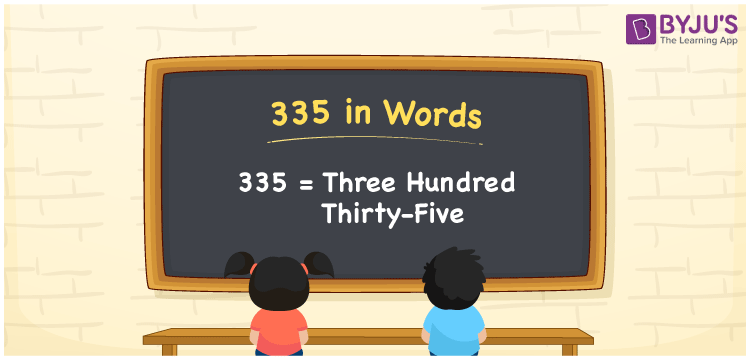# 335 in Words

The number 335 in words is “Three Hundred Thirty-five”. Learning the spelling of numbers is essential as it is helpful in our real-life activities, such as writing the number names in cheques, and so on. For converting the number 335 into words, we employ the place value system. Now, let us learn the spelling of 335 in words with a complete explanation.

 335 in Words: Three Hundred Thirty-five. Three Hundred Thirty-five in Numerical Form: 335.

## 335 in English Words## How to Write 335 in Words?

The following table shows the place values of the number 335:

 Hundreds Tens Ones 3 3 5

The expanded form of 335 is as follows:

= 3 × Hundred + 3 × Ten + 5 × One

= 3 × 100 + 3 × 10 + 5 × 1

= 300 + 30 + 5

= 335

= Three hundred thirty-five

Hence, 335 in words is three hundred thirty-five.

335 in words – Three hundred thirty-five

Is 335 an odd number? – Yes

Is 335 an even number? – No

Is 335 a perfect square number? – No

Is 335 a perfect cube number? – No

Is 335 a prime number? – No

Is 335 a composite number? – Yes

## Frequently Asked Questions on 335 in Words

Q1

### How to write 335 in words?

335 in words is three hundred thirty-five.

Q2

### Simplify 300 + 35, and express it in words.

Simplifying 300 + 35, we get 335. Hence, 335 in words is three hundred thirty-five.

Q3

### Is 335 an odd number?

Yes, 335 is an odd number.